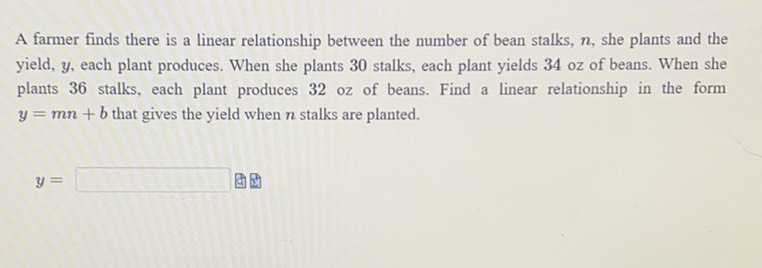### ¿Todavía tienes preguntas de matemáticas?

Pregunte a nuestros tutores expertos
Algebra
PreguntaA farmer finds there is a linear relationship between the number of bean stalks, $$n$$ , she plants and the yield, $$y$$ , each plant produces. When she plants $$30$$ stalks, each plant yields $$34$$ oz of beans. When she plants $$36$$ stalks, each plant produces $$32$$ oz of beans. Find a linear relationship in the form

$$y = m n + b$$ that gives the yield when $$n$$ stalks are planted.

$$y = 22 x + 360$$